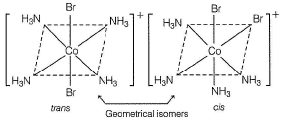# Retro (Past 13 Year) IIT-JEE Advanced (Coordination Compounds)

## 27 Questions MCQ Test | Retro (Past 13 Year) IIT-JEE Advanced (Coordination Compounds)

Description
This mock test of Retro (Past 13 Year) IIT-JEE Advanced (Coordination Compounds) for Class 12 helps you for every Class 12 entrance exam. This contains 27 Multiple Choice Questions for Class 12 Retro (Past 13 Year) IIT-JEE Advanced (Coordination Compounds) (mcq) to study with solutions a complete question bank. The solved questions answers in this Retro (Past 13 Year) IIT-JEE Advanced (Coordination Compounds) quiz give you a good mix of easy questions and tough questions. Class 12 students definitely take this Retro (Past 13 Year) IIT-JEE Advanced (Coordination Compounds) exercise for a better result in the exam. You can find other Retro (Past 13 Year) IIT-JEE Advanced (Coordination Compounds) extra questions, long questions & short questions for Class 12 on EduRev as well by searching above.
QUESTION: 1

### Match each coordination compound in Column I with an appropriate pair of characteristics from Column II and select the correct answer using the codes given below the columns (en = H2NCH2CH2NH2; atomic numbers : Ti = 22; Cr = 24; Co = 27; Pt = 78)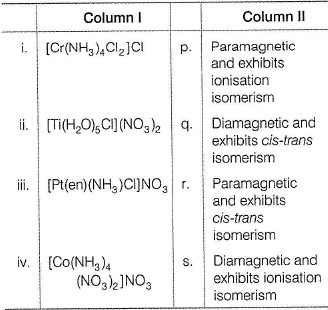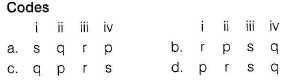(2014 Adv. Matching Type)

Solution:

Plan This problem is based on concept of VBT and magnetic properties of coordination compound.
Draw VBT for each coordination compounds.
If unpaired electron is present then coordination compound will be paramagnetic otherwise diamagnetic.
Coordination compounds of [MA4B2] type show geometrical isomerism.
Molecular orbital electronic configuration (MOEC) for various coordination compounds can be drawn using VBT as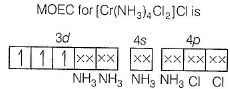Number of unpaired electrons (n) = 3
Magnetic property = paramagnetic Geometrical isomers of [Cr(NH3)4CI2]+ are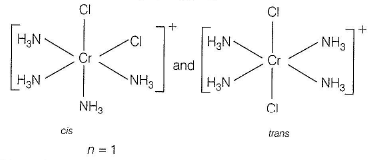Magnetic property = paramagnetic Ionisation isomers of [Ti(H2O)5CI](NO3)2 are
[Ti(H2O)5CI](NO3)2 and [Ti(H2O)5(NO3)]CI(NO3)
MOEC of [Pt(en)(NH3)CI]NO3 is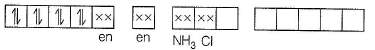n = 0
Magnetic property = diamagnetic Ionisation isomers are [Pt(en)(NH3)CI]NO3 and [Pt(en)NH3(NO3)]Cl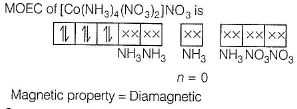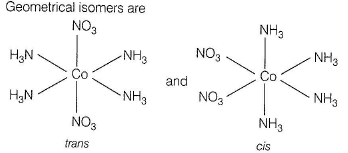Thus, magnetic property and isomerism in given coordination compounds can be summarised as
(i) [Cr(NH3)4CI2]Cl → Paramagnetic and exhibits cis-trans isomerism (r)
(ii) [Ti(H2O)5CI](NO3)→ Paramagnetic and exhibits ionisation isomerism (p)
(iii) [Pt(en)(NH3) CI]NO3 → Diamagnetic and exhibits ionisation isomerism (s)
(iv) [Co(NH3)4(NO3)2]NO3 → Diamagnetic and exhibits cis-trans isomerism (q)
(i) → (r), (ii) → (p), (iii) → (s), (iv) → (q)

*Answer can only contain numeric values
QUESTION: 2

### EDTA4- is ethylenediaminetetraacetate ion. The total number of N—Co—O bond angles in [Co(EDTA)]- complex ion is (2013Adv. Integer Type)

Solution:

Plan EDTA is a multidentate ligand as it can donate six pairs of electrons - two pair from the two nitrogen atoms and four pair from the four terminal oxygens of the —COO- groups.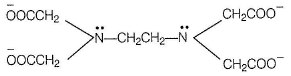The structure of a chelate of a divalent Co2+ with EDTA is shown as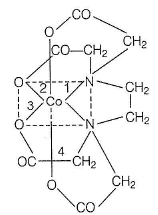Each N has four N—Co—O bonds thus, total eight N—Co—O bonds.

QUESTION: 3

### Consider the following complex ions, P, Q and R. P = [FeF6]3- Q = [V(H2O)6]2+ and R = [Fe(H2O)6]2+ The correct order of the complex ions, according to their spinonly magnetic moment values (in BM) is (2013 Adv. Only One Option Correct Type)

Solution:

Plan Spin only magnetic moment have the formula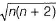BM, where n is the number of unpaired electrons . In presence of weak ligand (as H2O, Cl-, F-), there is no pairing of electrons and electrons donated by ligands are filled in outer vacant orbitals.
In presence of strong ligand (as CN-, CO, NH3, en), electrons are paired and electrons from ligands are filled in available inner orbitals.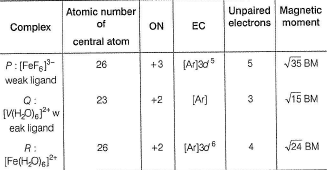Thus, order of spin only magnetic moment = Q < R < P

QUESTION: 4

The pair(s) of coordination complexes/ions exhibiting the same kind of isomerism is(are)

(2013 Adv. One or More than One Options Correct Type)

Solution:

ionisation isomers

QUESTION: 5

As per IUPAC nomenclature, the name of the complex [Co(H2O)4(NH3)2]CI3 is

(2012, Only One Option Correct Type)

Solution:

First of all, the compound has complex positive part [Co(H2O)4(NH3)2]3+ therefore , according to IUPAC conventions , positive part will be named first. Secondly , in writing name of complex , ligands are named first in alphabetical order irrespective of its charge , hence "ammine " will be written prior to “ aqua” .
Note In alphabetical order, original name of ligands are considered not the initials of prefixes. Also, special precaution should be taken in spelling name of NH3 ligand as it is ammine. Therefore, name ofthecomplex [Co(H2O)4(NH3)2]CI3 is Diamminetetraaquacobait? (III) chloride.

QUESTION: 6

NiCI2{P(C2H5)2(C6H5)}2 exhibits temperature dependent magnetic behaviour (paramagnetic/diamagnetic) the coordination geometries of Ni2+ in the paramagnetic and diamagnetic states respectively are

(2012, Only One Option Correct Type)

Solution:

In the given complex , NiCI2{P(C2H5)2(C6H5)}2 nickel is in + 2 oxidation state and the ground state electronic configuration of Ni2+ ion in free gaseous state is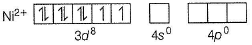For the given four coordinated complex to be paramagnetic , it must possess unpaired electrons in the valence shell. To satisfy this condition, four lone pairs from the four ligands occupies the four sp3 hybrid orbitals as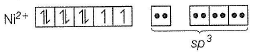There fore , geometry of paramagnetic complex must be tetrahedral. On the other hand , for complex to be diamagnetic, there should not be any unpaired electrons in the valence shell. This condition can be fullfilled by pairing electrons of 3d orbitals against Hund's rule as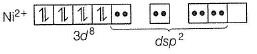The above electronic arrangement gives dsp2 hybridisation and therefore, square planar geometry of the complex.

QUESTION: 7

Geometrical shapes of the complexes formed by the reaction of Ni2+ with Cl-, CN- and H2O respectively, are

(2011, Only One Option Correct Type)

Solution:

Ni2+ + 4CN- → [Ni(CN)4]2-
Here, Ni2+ has d8 configuration with CN- as strong ligand.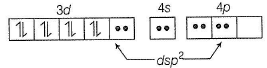d8 configuration in strong ligand field gives dsp2 hybridisation, hence square planar geometry.
Ni2+ + 4Cl- → [NiCI4]2-
Here, Ni2+ has d8 configuration with Cl- as weak ligand.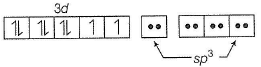d8 configuration in weak ligand field gives sp3 hydridisation, hence tetrahedral geometry.
Ni2+ with H2O forms [Ni(H2O)6]2+ complex and H2O is a weak ligand.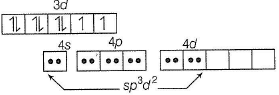Therefore, [Ni(H2O)6]2+ has octahedral geometry.

QUESTION: 8

Among the follow ing com plexes (K-P),

K3[Fe(CN)6] (K), [Co(NH3)6]CI3 (L) Na3[Co(ox)3] (M), [Ni(H2O)6]CI2 (N), K2 [Pt(CN)4](O) and [Zn(H2O)6] (NO3)2 (P) the diamagnetic complexes are

(2011, Only One Option Correct Type)

Solution:

For a diamagnetic complex , there should not be any unpaired electron in the valence shell of central metal.
In K3[Fe(CN)6], Fe (III) has d5-configuration (odd electrons), hence it is paramagnetic.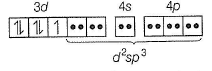In [Co(NH3)6]Cl3, Co (III) has d6-configuration in a strong ligand field, hence all the electrons are paired and the complex is diamagnetic.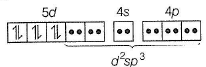In Na3[Co(ox)3], Co (III) has d6-configuration and oxalate being a chelating ligand, very strong ligand and all the six electrons remains paired in lower t2g level, diamagnetic.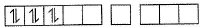In [Ni(H2O)6]CI2, Ni (II) has 3d8-configuration and H2O is a weak ligand hence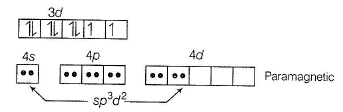In K2[Pt(CN)4], Pt(ll) has d8 configuration and CN- is a strong ligand, hence all the eight electrons are spin-paired. Therefore, complex is diamagnetic.
In [Zn(H2O)6] (NO3)2 Zn (II) has 3d10 configuration with all the ten electrons spin paired, hence diamagnetic.

*Answer can only contain numeric values
QUESTION: 9

The volume (in mL) of 0.1 M AgNO3 required for complete precipitation of chloride ions present in 30 mL of 0.01 M solution of [Cr(H2O)5CI]CI2, as Silver Chloride is Close to

(2011, Integer Type)

Solution:

mmol of complex = 30 x 0.01 = 0.3
Also, 1 mole of complex [Cr(H2O)5CI]CI2 gives only two moles of chloride ion when dissolved in solution.
[Cr(H2O)5CI]CI→ [Cr(H2O)5CI]2+ + 2Cl-
mmol of Cl- ion produced from its 0.3 mmol = 0.6
Hence, 0.6 mmol of Ag+ would be required for precipitation.
0.60 mmol of Ag+ = 0.1 M x V(in mL)
V = 6mL

*Answer can only contain numeric values
QUESTION: 10

Total number of geometrical isomers for the complex [RhCI(CO)(PPh3)(NH3)] is

(2010, Integer Type)

Solution: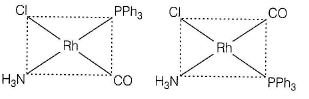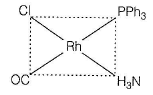3 isomers.

QUESTION: 11

The ionisation isomer of [Cr(H2O)4CI(NO2)]CI is

(2010, Only One Option Correct Type)

Solution:

Ionisation isomers are the complexes that produces different ions in solution, i.e. they have ions interchanged inside and outside the coordination sphere.
[Cr(H2O)4CI(NO2)]CI and [Cr(H2O)4CI2] (NO2) have different ions inside and outside the coordinate sphere and they are isomers. Therefore, they are ionisation isomers.

QUESTION: 12

The correct structure of ethylenediaminetetraacetic acid (EDTA) is

(2010, Only One Option Correct Type)

Solution: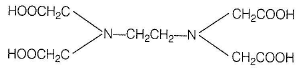QUESTION: 13

The Complex showing a spin-only magnetic moment of 2.82 B

(2010, Only One Option Correct Type)

Solution:

Magnetic moment = 2.83 BM indicates that there is two unpaired electrons.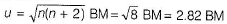In [NiCl4]2-, Ni has d8 configuration and Cl- is a weak ligand: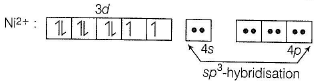*Multiple options can be correct
QUESTION: 14

The Compound(s) that exhibit(s) geometrical isomerism is(are)

(2009, One or More than One Options Correct Type)

Solution:

Both [Pt(en)2CI2]CI2 and [Pt(NH3)2CI2] are capable of showing geometrical isomerism.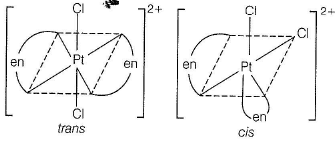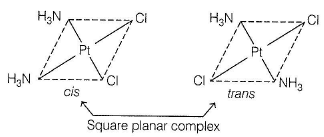QUESTION: 15

The spin only magnetic moment value (in Bohr magneton units) of Cr(CO)6 is

(2009, Only One Options Correct Type)

Solution:

In Cr(CO)6, 3d6, has no unpaired electrons, zero magnetic moment because CO is strong field ligand.

QUESTION: 16

Both [Ni(CO)4] and [Ni(CN)4]2- are diamagnetic. The hybridisation of nickel in these complexes respectively, are

(2008, Only One Option Correct Type)

Solution:

In Ni(CO)4, Ni is sp3 hybridised while in [Ni(CN)4]2-, Ni2+ is dsp2 hybridised because CO is strong field ligand.

QUESTION: 17

The IUPAC name of [Ni(NH3)4][NiCI4] is

Solution:

[Ni(NH3)4]2+ = tetraamminenickel (II)
[NiCI4]2- = tetrachloronickelate (II)
Cationic part is named first, hence tetraamminenickel (ll)-tetrachloronickelate(ll)

QUESTION: 18

Among the following, the coloured compound is

Solution:

CuF2:Cu2+ has 3d9 configuration allowed d-d transition.hence coloured.

QUESTION: 19

Direction : This section is based on Statement I and Statement II. Select the correct answer from the codes given below.

Q.

Statment I : The geometrical isomers of the complex [M(NH3)4CI2]are optically inactive.

Statment II : Both geometrical isomers of the complex [M(NH3)4CI2] possess axis of symmetry.

Solution:

Both statements are true. However, axis of symmetry is not a criteria of optical isomerism. Optical inactivity of the two geometrical isomers of [M(NH3)4CI2] is due to the presence of plane of symmetry.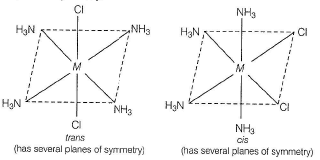QUESTION: 20

Statement I : [Fe(H2O)5NO]SO4 is paramagnetic.

Statement II : Fe in [Fe(H2O)5NO]SO4 has three unpaired electrons.

(2008, Statement Type)

Solution:

In the complex [Fe(H2O)5NO]SO4, Fe is in + 1 oxidation state because NO is in +1 state. Also, NO is a strong ligand, complex has 3d7 configuration at Fe(l) as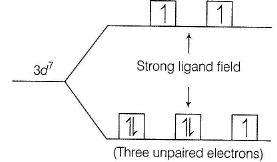QUESTION: 21

Match the complexes in Column I with their properties listed in Column II.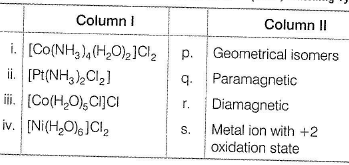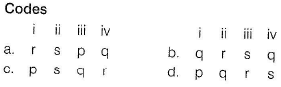(2007, Matching Type)

Solution:

(i) [Co(NH3)4(H2O)2]CI2 : Co2+, 3d7
show geometrical isomerism, paramagnetic.
(ii) Pt(NH3)2CI2 : Pt2+ has d8 configuration with all paired electrons.
Show geometrical isomerism, diamagnetic.
(iii) [Co(H2O)5CI]CI : Co2+, 3d7
cannot show geometrical isomerism, paramagnetic.
(iv) [Ni(H2O)6]CI2 : Ni2+ , 3d8 , weak ligand, has two unpaired electrons. Paramagnetic but cannot show geometrical isomerism.
(i) → (r), (ii) → (p), (iii) → (s), (iv) → (q)

QUESTION: 22

Among the following metal carbonyls,the  C—O bond order is lowest in

(2007, Only One Option Correct Type)

Solution:

Greater the extent of dπ-pπ back bonding, smaller will be the bond order of CO bond in metal carbonyls. In Fe(CO)5, there is maximum number of valence shell electrons (d-electrons), greatest chances of pπ-dπ back bonding, lowest bond order of CO bond.

QUESTION: 23

If the bond length of CO bond in carbon monoxide is 1.128 Å, then what is the value of CO bond length in Fe(CO)5 ?

Solution:

In CO, bond order = 3. In metal carbonyls like Fe(CO)5, due to dπ-pπ back bonding, bond order of CO decreases slightly therefore, bond length increases slightly,

QUESTION: 24

Comprehension Type

This section contains a passage describing theory, experiments, data, etc. Two questions related to the paragraph have been given. Each question has only one correct answer out of the given 4 options (a), (b), (c) and (d)

The coordination number of Ni2+ is 4.
NiCI2 + KCN (excess) → A (cyano complex)
NiCI2 + conc. HCI (excess) → B (chloro complex)

Q.

The IUPAC name of A and B are

(2006, Comprehension Type)

Solution:

A = K2[Ni(CN)4]; B = K2[NiCI4]
A : Potassium tetracyanonickelate (II)
B : Potassium tetrachloronickelate (II)

QUESTION: 25

The coordination number of Ni2+ is 4.
NiCI2 + KCN (excess) → A (cyano complex)
NiCI2 + conc. HCI (excess) → B (chloro complex)

Q.

Predict the magnetic nature of A and B.

Solution:

A is diamagnetic, square planar complex because of strong ligand field ofCN-.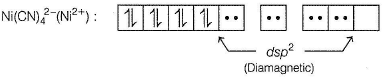B is paramagnetic, tetrahedral complex because of weak ligand field ofCl-.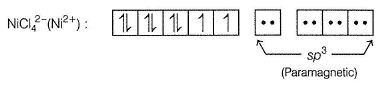QUESTION: 26

The coordination number of Ni2+ is 4.
NiCI2 + KCN (excess) → A (cyano complex)
NiCI2 + conc. HCI (excess) → B (chloro complex)

Q.

The hybridisation of A and B

Solution:

Described in sol.25, A has dsp2 hybridisation while B has sp3 hybridisation of Ni.

QUESTION: 27

Which kind of isomerism is shown by Co(NH3)4Br2Cl ?

(2005, Only One Option Correct Type)

Solution:

[Co(NH3)4Br2]CI and [Co(NH3)4BrCI]Br are ionisation isomers.